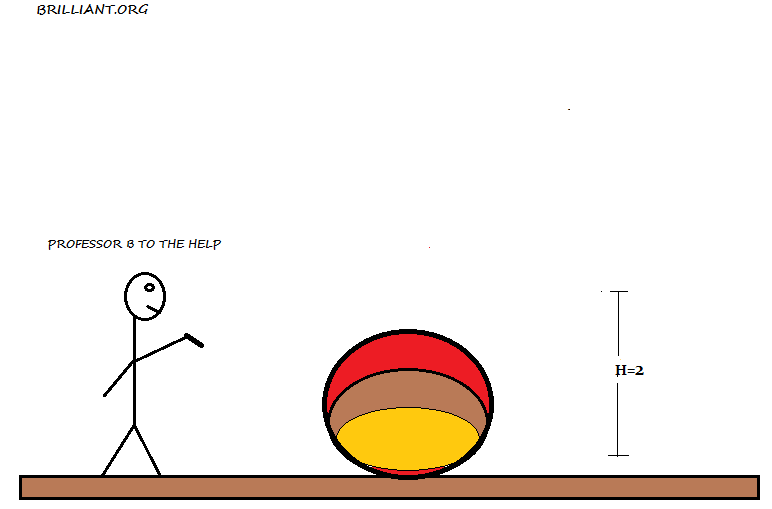# How long will you collide?A ball of mass $1\textrm{ kg}$ is dropped from a height $H=2\textrm{ m}$. Estimate how long the ball is in contact with the ground (in seconds)?

Details and assumptions

• Simplify the question by modeling the ball as an ideal spring of spring constant $100 \textrm{ Nm}|$.
• If necessary, take the gravitational acceleration to be $9.8\textrm{ m/s}^2$.
×